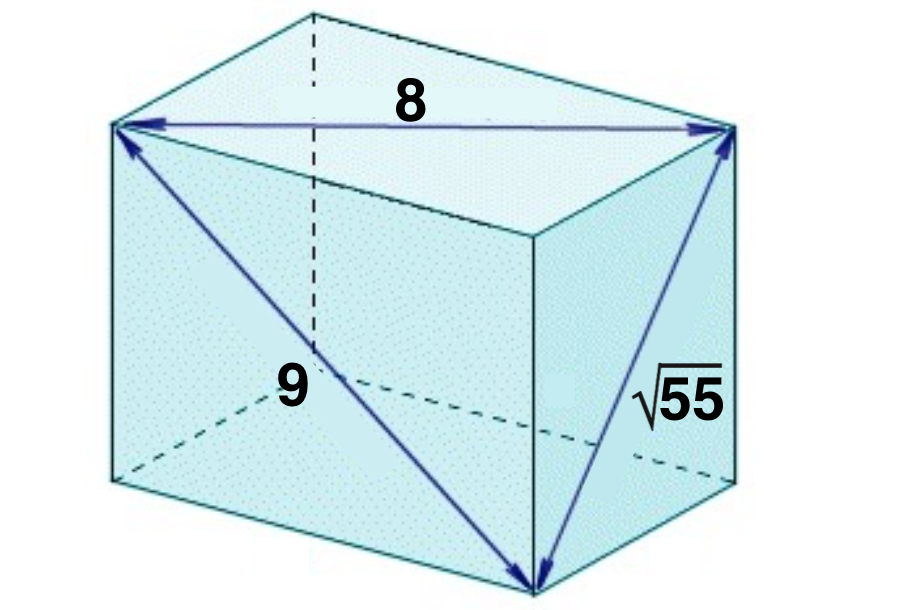# Day 10: Diagonals of a Cuboid

Geometry Level 2The three face diagonals (shown in the diagram) of a cuboid are of length $\sqrt{55}, 8$ and $9$.

Find the length of the longest diagonal in the cuboid.

×